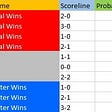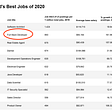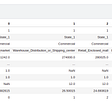# How To Detect Outliers In Dataset

Detect and Handle the outliers is biggest and challengeable task in Machine learning.

Outliers directly effect on model accuracy.

First let understand , what is the outliers in dataset?

An outlier is a data set that is distant from all other observations. A data points that lies outside the overall distribution of the dataset.

Now, let understand with the help of example….

In an organization, The salary range of all employees in between 10k\$ to 50k\$.

So, in salary column all employee’s salaries fall under the given range.

Suppose, we have 10 employees in an organization and their salaries distributions.

These all the list of employee’s salaries. so it’s clearly visible 1,50,000\$ is not in range and it doesn’t fall in between 10k\$ to 50k\$. So, It indicates outlier of this salary column.

Outliers occurs by human errors like wrong entry ,Variability in the data and an experimental measurement error etc. but it might be possible in our case the salary of CEO is 1,50,000\$. How can you say this done by human mistake.

In our case, there were only 10 entries and we could easily find outlier manually or by hand or by watch .but if we have millions of entries so that time how will you find out the outlier from million entries.

## There are some majorly used techniques. we will discussed later..

1-Using scatter plots.

2-Using Box plot.

3-Using z score.

4-Using the IQR interquartile range.

We will discuss all of these in detail….

# What are the impacts of having outliers in a dataset?

1. It causes various problems during our statistical analysis.
2. It may cause a significant impact on the mean and the standard deviation.
3. It directly effect on the model’s accuracy.

# 1. Detecting outlier using Scatter Plot

`import matplotlib.pyplot as pltx = [5,7,8,7,2,17,2,9,4,9,8,9,6]y = [86,86,87,88,111,86,103,87,88,81,80,85,86]plt.scatter(x, y)plt.show()`

x and y are our data points and right here we are trying to find out the outliers from the data using scatter plot.

Right here, clearly visible three outliers in dataset. These three dots is not in range of data points variability.

# Using Z score

Formula for Z score = (Observation — Mean)/Standard Deviation

z = (X — μ) / σ

Data point that falls outside of 3 standard deviations. we can use a z score and if the z score falls outside of 2 standard deviation.

`outliers=[]dataset=[11,10,12,14,12,15,14,13,15,102,12,14,17,19,107,10,13,12,14,12,108,12,11,14,13,15,10,15,12,10,14,13,15,10]def detect_outliers(data):        threshold=3    mean = np.mean(data)    std =np.std(data)            for i in data:        z_score= (i - mean)/std         if np.abs(z_score) > threshold:            outliers.append(y)    return outliersoutlier_pt=detect_outliers(dataset)print(outlier_pt)`

These three [102,107,108] are outliers.

# 3. Detecting outliers using InterQuantile Range

75%- 25% values in a dataset

## 5.Find upper bound q3*1.5

Anything that lies outside of lower and upper bound is an outlier.

`dataset= [11,10,12,14,12,15,14,13,15,102,12,14,17,19,107, 10,13,12,14,12,108,12,11,14,13,15,10,15,12,10,14,13,15,10]import numpy as npdataset= [11,10,12,14,12,15,14,13,15,102,12,14,17,19,107, 10,13,12,14,12,108,12,11,14,13,15,10,15,12,10,14,13,15,10]quantile1, quantile3= np.percentile(dataset,[25,75])print("range between quantile1 to quantile3")print(quantile1,quantile3)print("IQR")iqr_value=quantile3-quantile1print(iqr_value)print("Find the lower bound value and the higher bound value")lower_bound_val = quantile1 -(1.5 * iqr_value)upper_bound_val = quantile3 +(1.5 * iqr_value)print(lower_bound_val,upper_bound_val)`

SO, Data points below 7.5 and above 19.5 consider as outliers.

# 4. Detecting the outliers using Box Plots

Draw a box plot on given dataset and detect the outliers using box plots.

`import matplotlib.pyplot as pltvalue1 = [82,76,24,40,67,62,75,78,71,32,98,89,78,67,72,82,87,66,56,52]box_plot_data=[value1]plt.boxplot(box_plot_data)plt.show()`

These are four most commonly used techniques to detect the outliers from the datasets.

In next tutorial , we will discuss about how we can handle outliers.

--

--

--

## More from Puneet Singh

Data Science , Machine Learning , BlockChain Developer

Love podcasts or audiobooks? Learn on the go with our new app.

## A Monte Carlo Simulation of the 2017–18 Premier League Season## Decorators and Closures by Example in Python## A REVOLUTION is unfolding all around us.## Linear regression using Apache Spark MLlib — Wisdom In Data## A/B Testing with Heterogeneous Treatment## Scaling Access to Top Quality Doctors: Our Investment in Garner Health## Puneet Singh

Data Science , Machine Learning , BlockChain Developer

## Predicting system behavior and anticipating anomalies using python and machine learning## Predicting Student Performance Using Machine Learning## Approaching Kaggle Competition step by step par## Machine learning scholar adventure: Chapter 5